Learn US

# Breaking down the myth of "Is Trigonometry Hard?"

1.2k views

 1 Introduction 2 Is Trigonometry hard? 3 How do we do Trigonometry easily then? 4 Summary 5 External References

09 September 2020

## Introduction

Trigonometry skills play an important role in a wide variety of careers, including architecture and engineering.

Because of this, it’s important for students that are interested in the scientific or engineering fields to understand trigonometry.

So answering the common doubt "Is trigonometry hard?" is very crucial for students.Trigonometry is simply the study of triangles – more specifically, the study of the angles and dimensions of triangles.

Although this sounds quite trivial, trigonometry is a necessary and important part of modern engineering, navigation, design, architecture, and other fields.

## Is Trigonometry hard?

But why do students find it hard then?

The answer lies in the fact that you are not able to relate and appreciate the importance of trigonometry in real life. It is limited to academic importance only. So this results in a lack of interest and effort. This slowly turns trigonometry into a mini monster.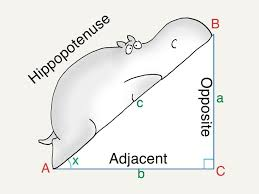But it is neither hard nor impossible. Everything depends upon us. Mathematics is one of the best subjects where we can understand various real-life phenomenons and have an idea of the real world around us. If you think trigonometry is hard then first of all you should start by concentrating on it. In trigonometry, there are three main things that can be broken down into, which are sine, cos, and tan.

If you understand these three things, you will feel that trigonometry is basically nothing and that means it gives newfound energy to solve other problems. So don't worry about trigonometry and have fun with mathematics along with trigonometry. So be positive and optimistic about trigonometry.

Use trigonometry cheat sheets for solving problems and quick results. But there is no substitute for trigonometry practice. The more effort one puts in, the more confident one gets with Trigonometry formulas, functions. For the Trigonometry cheat sheet, visit Trigonometric Cheat Sheet.

Trig formulas, functions can be customized in mnemonics like SOHCAHTOA to feed in our memory..
I think you deserve a little it more, and here’s a glimpse of what made trig click for me.

• Imagine a dome, a wall, and a ceiling or real-life scenarios.
• Trig functions are percentages to these shapes and can be applied to all these for architecture and design purposes.

Some Trigonometry real-life examples:

In summer if you going out, you always try to keep yourself within the shade ( a tree or a building) but have you ever observed that in day time the shadow is small in size and at morning or evening shadow is large in size why this is happening, the size of building or tree is not changing even the sun's size is not changing?The reason we know this is that the position of the sun is changing with respect to the earth.
This change in position is explained by the angles and their respective trigonometric ratios. As the angle changes from 0º to 180º, the shadow size changes since the trigonometric lengths with respect to these angles change, and at 90º the position of the sun is exactly above the object that's why their shadow becomes diminished. From 0º to 180º, the shadow starts first decreasing till 90º and then it starts increasing in size again.

Let's take examples of Trigonometry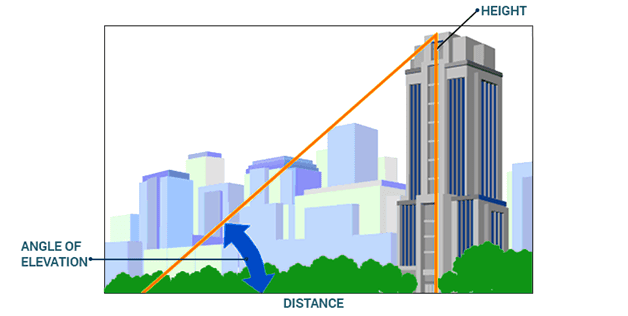In the given figure if know the elevation angle and distance of the building you can easily calculate the height of the building using, tan theta = h/d

Electric poles are present everywhere. They are usually erected by using a  wire. The pole, wire, and the ground form a triangle. But, if the length of the wire is short, what will be the triangle shape, and what will be the angle of the wire with the ground?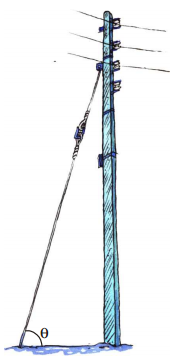A person is painting a wall with the help of a stair which is kept as shown in the adjacent figure below. If the man wants to paint at a higher position, what will the man do?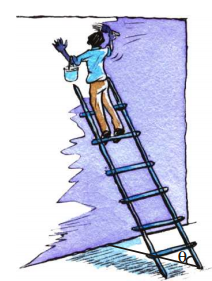In a park, children like to slide on sliders on a defined angle from the earth. What will happen to the slider if we change the angle? Are children still able to play on it?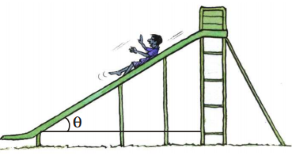The above examples are geometrically showing the application of triangles in our daily life and we can measure the heights, distances, and slopes by using the properties of triangles. These types of problems are part of ‘trigonometry’ which is a branch of mathematics.
Now let us explore the example of a person who is whitewashing the wall with the help of a ladder as shown in a previous figure. We observe some conditions.
We denote the foot of the ladder by A and the top of it by C and the point of joining height of the wall and base of the ladder as B. Therefore, ∆ABC is a right angle triangle with right angle at B. The angle between the ladder and base is said to be θ.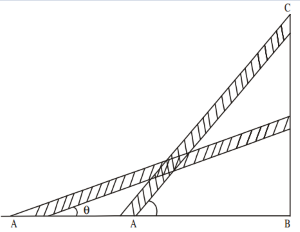If the person wants to paint at a higher point on the wall-
● What happens to the angle made by the ladder with the ground?
● What is the change in the distance AB?

If the person wants to whitewash at a lower point on the wall-
● What happens to the angle made by the ladder with the ground?
● What is the change in the distance AB?

So, when ‘θ’ is increased, the height also increases, and the base decreases. But, when θ is decreased, the height also decreases, and the base increases.

Here, we have seen a right angle triangle ABC and have given ordinary names to all sides and angles.

## How do we do Trigonometry easily then?

To be able to do Trignometry properly, one must be sure of the basic concept, should have enough trigonometry problems practice, and should have it clear in their head a step by step guide on how to approach the questions.

To learn how to approach questions and solve trigonometry questions do visit Trigonometry Cheat Sheet

And to understand in depth about trigonometry problems and how to solve hard trigonometry questions, visit Trigonometry Problems

Here are some additional points that talk about Breaking down the myth of "Is Trigonometry Hard?". To view them click on the Download button.

## Summary

Trigonometric functions are used to determine properties of any angle, relationships in any triangle, and the graphs of any recurring cycle.

So knowing how to do trigonometry is very essential.

Learning trigonometry helps you understand, visualize, and graph these relationships and cycles.

If you are studying on your own with staying attentive in class, you get to know the basic trigonometric concepts and likely start noticing cycles in the world around you by generalizing all the concepts which you have learned so far.

Written by Gargi Shrivastava, Cuemath Teacher